#### How to Calculate Loan Amortization in Excel

You might already be familiar with the loan payment system. It is obvious that your loan principal decreases with each loan payment you make over the life of your loan. The loan payment you make does not fully contribute towards your principal amount.

Instead, a portion of your payment goes towards your principal and the remaining portion goes towards the interest of the loan. The process by which your loan principal decreases over the life of your loan is known as amortization.

Before taking a loan, you should be very clear about the total amount you need to pay to pay off the loan (principal + interest), the loan payment per installment, the number of payments, the amount you pay towards principal and the loan, and so on.

Excel offers a number of financial functions that help you calculate amortization easily.

## Calculate the Amortization

Open Excel and save the file as amortization.xlsx. Type “Loan Amount” in A1, “Loan Term (Years)” in A2, “Payments Per Year” in A3, “Annual Interest Rate” in A4 and “One Time Payment” in A5. Also type “Period” in A7, “Beginning Balance” in B7, “Payment” in C7, “Principal” in D7, “Interest” in E7, and “Ending Balance” in F7.

You should not enter the double quotes when you type in the data. You can format these texts and make it bold.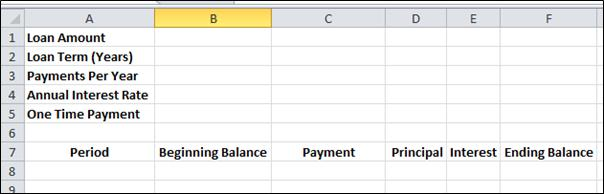Enter the details of your loan in cells B1 to B4, loan amount in B1 (say 20,000), loan term in years in B2 (say 1.5), number of payments per year (say 12) in B3 and interest rate in B4 (say 7). You can format the cells B1 and B5 to include your preferred currency. Right-click the cell B1 and select Format Cells.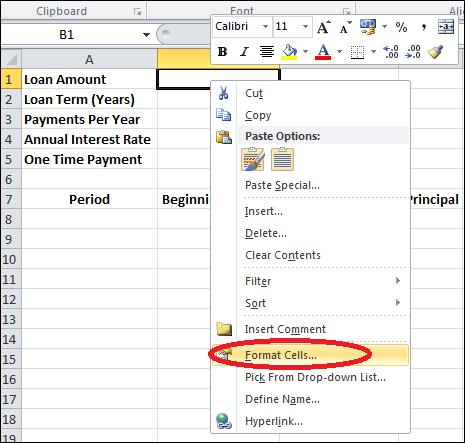You will get a window and select Currency from the Category and choose your preferred currency.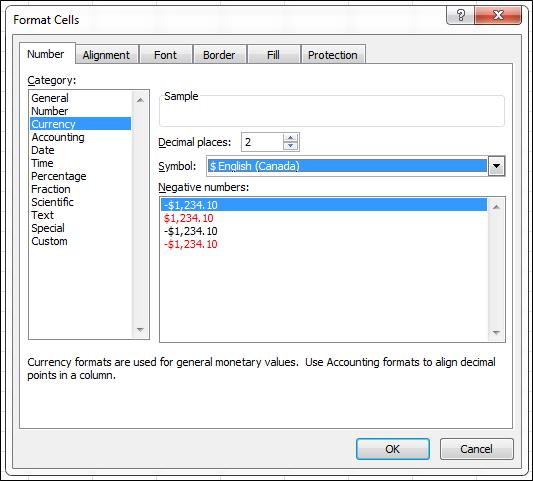Format cell B4 to include a percentage. Instead of Currency, choose Percentage from the Category.

### PMT formula

Next, you’ll need to calculate the monthly payment. To do this, you can use the PMT function in Excel. The PMT function calculates the payment for a loan based on constant payments and a constant interest rate. The syntax for the PMT function is:

=PMT(rate, nper, pv, [fv], [type])

where:

• rate = annual interest rate divided by the number of payment periods
• nper = total number of payment periods
• pv = loan amount
• [fv] = future value of the loan (optional)
• [type] = payment type (0 for end of period, 1 for start of period; optional)

So, for a loan with a \$20,000 principal, a 7% annual interest rate, and a loan term of 18 months, the formula to calculate the monthly payment would be:

=PMT(7%/12, 12*1.5, -20000)

Click the cell B5 and in the formula bar and enter the formula =PMT(B4/12,B3*B2,-B1).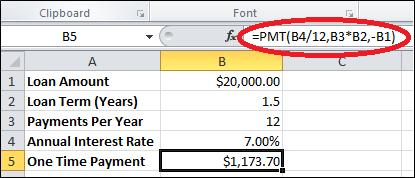You could find that the amount you need to pay per installment is \$1,173.7.

As your loan period is 1.5 years (18 months), enter 1 to 18 in cells A8 to A25. Format the cells B8 through F25 to contain currency by following the steps explained earlier.

### PPMT and IPMT formulas

In Microsoft Excel, you can calculate loan amortization using the PPMT (Payment) and IPMT (Interest Payment) functions.

1. Calculate the payment amount: Use the following formula to calculate the payment amount: =PPMT(interest rate, period number, total number of periods, loan amount)
2. Calculate the interest payment: Use the following formula to calculate the interest payment for each period: =IPMT(interest rate, period number, total number of periods, loan amount, 0)
3. Set up an amortization schedule: To set up an amortization schedule, use the PPMT and IPMT formulas in consecutive cells to calculate the payment amount and interest payment for each period.
4. Calculate the principal payment: To calculate the principal payment, subtract the interest payment from the payment amount.
5. Update the remaining loan balance: To update the remaining loan balance, subtract the principal payment from the previous balance.
6. Repeat the process: Repeat the process for each period until the loan is fully paid off.

The beginning balance at the start of the loan period will be the loan amount. So, enter “=B1” in cell B8 and press Enter key. In cell C8, enter =\$B\$5 and press Enter as it is the one time payment (payment per installment).

Click cell D8 and enter =PPMT(\$B\$4/12,A8,\$B\$2*\$B\$3,-\$B\$1).

Click cell E8 and enter =IPMT(\$B\$4/12,A8,\$B\$2*\$B\$3,-\$B\$1).

Click cell F8 and enter =B8-D8.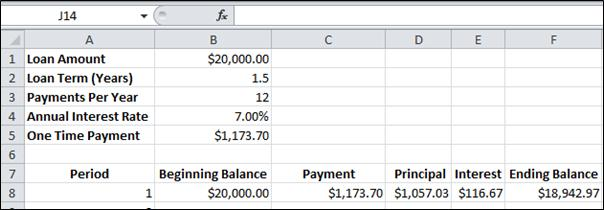Next you need to set the beginning balance for the second period. Of course, the begging balance of the second period would be the ending balance of first period. Click cell A9 and enter “=F8” and press Enter. Copy the formulas from C8 to F8 and paste them in C9 to F9.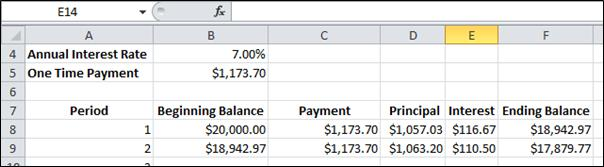Select cells A9 through F9, mouse over the bottom right corner of the selection to receive a crosshair cursor and then click and drag the selection down to row 25.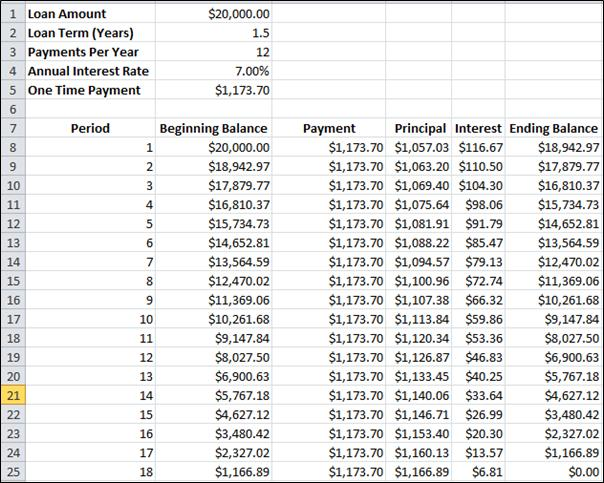Now the amortization table is completed.

Once you have calculated the loan amortization in Excel, you can analyze the results to get a better understanding of how your loan is being paid off. Here are some steps you can follow:

1. Review the payment amount: Look at the payment amount and see if it is within your budget. If the payment is too high, consider adjusting the loan term or interest rate to lower the payment.
2. Analyze the interest and principal payments: Look at the breakdown of each payment into interest and principal. In the early stages of the loan, the majority of the payment will be applied to interest, while in later stages, the majority of the payment will be applied to the principal.
3. Track the remaining balance: Observe the remaining balance over time to see how it decreases with each payment.
4. Compare the amortization schedule to other loans: If you have taken out multiple loans, you can compare the amortization schedules to see which loan is being paid off more efficiently.
5. Determine the impact of extra payments: Consider what would happen if you made additional payments on your loan. Use the Excel amortization schedule as a tool to see how extra payments would impact your loan balance and the overall cost of the loan.